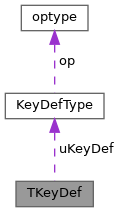ReactOS 0.4.15-dev-6067-g0b695a6
TKeyDef Class Reference

`#include <tkeydef.h>`

Collaboration diagram for TKeyDef:[legend]

## Public Member Functions

TKeyDef ()

TKeyDef (char *def, DWORD state, DWORD code)

TKeyDef (optype op, DWORD state, DWORD code)

TKeyDef (const TKeyDef &t)

charoperator= (char *def)

DWORD operator= (DWORD code)

TKeyDefoperator= (const TKeyDef &t)

const optypeoperator= (optype op)

~TKeyDef ()

const charGetszKey ()

const KeyDefType GetKeyDef ()

DWORD GetCodeKey ()

## Private Attributes

KeyDefType uKeyDef

DWORD vk_code

DWORD dwState

## Friends

bool operator== (const TKeyDef &t1, const TKeyDef &t2)

bool operator< (const TKeyDef &t1, const TKeyDef &t2)

## Detailed Description

Definition at line 36 of file tkeydef.h.

## ◆ TKeyDef() [1/4]

 TKeyDef::TKeyDef ( )

Definition at line 37 of file tkeydef.cpp.

37 {
38 uKeyDef.szKeyDef = 0;
39 vk_code = dwState = 0;
40}
DWORD dwState
Definition: tkeydef.h:40
DWORD vk_code
Definition: tkeydef.h:39
KeyDefType uKeyDef
Definition: tkeydef.h:38
char * szKeyDef
Definition: tkeydef.h:27

## ◆ TKeyDef() [2/4]

 TKeyDef::TKeyDef ( char * def, DWORD state, DWORD code )

Definition at line 42 of file tkeydef.cpp.

42 {
43 uKeyDef.szKeyDef = 0;
44 if (def != NULL && *def != 0) {
45 // szKeyDef = (char *) GlobalAlloc(GPTR, strlen(def) +1);
46 uKeyDef.szKeyDef = new char[strlen(def)+1];
48 }
49 dwState = state;
50 vk_code = code;
51}
ACPI_SIZE strlen(const char *String)
Definition: utclib.c:269
char * strcpy(char *DstString, const char *SrcString)
Definition: utclib.c:388
static int state
Definition: maze.c:121
#define NULL
Definition: types.h:112
Definition: inflate.c:139

## ◆ TKeyDef() [3/4]

 TKeyDef::TKeyDef ( optype op, DWORD state, DWORD code )

Definition at line 53 of file tkeydef.cpp.

53 {
54 uKeyDef.op = new optype;
55 uKeyDef.op->sendstr = 0;
56 uKeyDef.op->the_op = op.the_op;
57 dwState = state;
58 vk_code = code;
59}
UINT op
Definition: effect.c:236
Definition: tkeydef.h:21
tn_ops the_op
Definition: tkeydef.h:23
char sendstr
Definition: tkeydef.h:22
optype * op
Definition: tkeydef.h:28

## ◆ TKeyDef() [4/4]

 TKeyDef::TKeyDef ( const TKeyDef & t )

Definition at line 61 of file tkeydef.cpp.

61 {
62 if(t.uKeyDef.szKeyDef == NULL) {
63 uKeyDef.szKeyDef = (char *)NULL;
64 } else if(t.uKeyDef.op->sendstr == 0) {
65 uKeyDef.op = new optype;
66 uKeyDef.op->sendstr = 0;
67 uKeyDef.op->the_op = t.uKeyDef.op->the_op;
68 } else {
69 uKeyDef.szKeyDef = new char[strlen(t.uKeyDef.szKeyDef)+1];
70 strcpy(uKeyDef.szKeyDef, t.uKeyDef.szKeyDef);
71 }
72 dwState = t.dwState;
73 vk_code = t.vk_code;
74}
GLdouble GLdouble t
Definition: gl.h:2047

## ◆ ~TKeyDef()

 TKeyDef::~TKeyDef ( )

Definition at line 76 of file tkeydef.cpp.

76 {
77 if(uKeyDef.szKeyDef) delete[] uKeyDef.szKeyDef;
78}

## ◆ GetCodeKey()

 DWORD TKeyDef::GetCodeKey ( )
inline

Definition at line 66 of file tkeydef.h.

66{ return vk_code; }

## ◆ GetKeyDef()

 const KeyDefType TKeyDef::GetKeyDef ( )
inline

Definition at line 65 of file tkeydef.h.

65{ return uKeyDef; }

## ◆ GetszKey()

 const char * TKeyDef::GetszKey ( )
inline

Definition at line 64 of file tkeydef.h.

64{ return uKeyDef.szKeyDef; }

## ◆ operator=() [1/4]

 char * TKeyDef::operator= ( char * def )

Definition at line 80 of file tkeydef.cpp.

80 {
81 if(def != NULL && *def != 0) {
82 if(uKeyDef.szKeyDef) delete[] uKeyDef.szKeyDef;
83 uKeyDef.szKeyDef = new char[strlen(def)+1];
85 }
86 return uKeyDef.szKeyDef;
87}

## ◆ operator=() [2/4]

 TKeyDef & TKeyDef::operator= ( const TKeyDef & t )

Definition at line 93 of file tkeydef.cpp.

93 {
94 if(t.uKeyDef.szKeyDef) {
95 if(uKeyDef.szKeyDef) delete[] uKeyDef.szKeyDef;
96 if(t.uKeyDef.op->sendstr) {
97 uKeyDef.szKeyDef = new char[strlen(t.uKeyDef.szKeyDef)+1];
98 strcpy(uKeyDef.szKeyDef, t.uKeyDef.szKeyDef);
99 } else {
100 uKeyDef.op = new optype;
101 uKeyDef.op->sendstr = 0;
102 uKeyDef.op->the_op = t.uKeyDef.op->the_op;
103 }
104 } else {
105 uKeyDef.szKeyDef = (char *)NULL;
106 }
107 dwState = t.dwState;
108 vk_code = t.vk_code;
109 return *this;
110}

## ◆ operator=() [3/4]

 DWORD TKeyDef::operator= ( DWORD code )

Definition at line 89 of file tkeydef.cpp.

89 {
90 return vk_code = code;
91}

## ◆ operator=() [4/4]

 const optype & TKeyDef::operator= ( optype op )

Definition at line 112 of file tkeydef.cpp.

112 {
113 uKeyDef.op = new optype;
114 uKeyDef.op->sendstr = 0;
115 uKeyDef.op->the_op = op.the_op;
116 return *uKeyDef.op;
117}

## ◆ operator<

 bool operator< ( const TKeyDef & t1, const TKeyDef & t2 )
friend

Definition at line 126 of file tkeydef.cpp.

126 {
127 if (t1.vk_code == t2.vk_code) return t1.dwState < t2.dwState;
128 return t1.vk_code < t2.vk_code;
129}

## ◆ operator==

 bool operator== ( const TKeyDef & t1, const TKeyDef & t2 )
friend

Definition at line 122 of file tkeydef.cpp.

122 {
123 return ((t1.vk_code == t2.vk_code) && (t1.dwState == t2.dwState));
124}

## ◆ dwState

 DWORD TKeyDef::dwState
private

Definition at line 40 of file tkeydef.h.

Referenced by operator=(), and TKeyDef().

## ◆ uKeyDef

 KeyDefType TKeyDef::uKeyDef
private

Definition at line 38 of file tkeydef.h.

Referenced by GetKeyDef(), GetszKey(), operator=(), TKeyDef(), and ~TKeyDef().

## ◆ vk_code

 DWORD TKeyDef::vk_code
private

Definition at line 39 of file tkeydef.h.

Referenced by GetCodeKey(), operator=(), and TKeyDef().

The documentation for this class was generated from the following files: